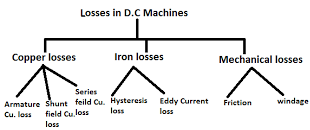## Types of Losses in D.C. Machines:

The losses in d.c machines (generator or motor) may be divided into three classes viz

(i) Copper losses

(ii) Iron or core losses

(iii) Mechanical losses

All these losses appear as heat and thus raise the temperature of the machine.They also lower the efficiency of the machine.### 1.Copper losses:

These losses in dc machines occur due to currents in the various windings of the machine.

(i) Armature copper loss = a 2 a RI

(ii) Shunt field copper loss = sh 2 sh RI

(iii) Series field copper loss = se 2 se RI

Note: There is also brush contact loss due to brush contact resistance (i.e., the resistance between the surface of brush and surface of commutator). This loss is generally included in armature copper loss.

### 2.Iron or Core losses:

These losses in dc machines occur in the armature of a d.c machine and are due to the rotation of armature in the magnetic field of the poles.They are of two types viz., (i) Hysteresis losses (ii) Eddy current losses.

(i) Hysteresis loss: Hysteresis loss occurs in the armature of the d.c machine since any given part of the armature is subjected to magnetic field reversals as it passes under successive poles.The figure shows an armature rotating in a two-pole machine.Consider a small piece ab of the armature. When the piece ab is under N-pole, the magnetic lines pass from a to b. Half a revolution later, the same piece of iron is under S-pole and magnetic lines pass from b to a so that magnetism in the iron is reversed. In order to reverse continuously the molecular magnets in the armature core, some amount of power has to be spent which is called Hysteresis Losses. It is given by Steinmetz formula. In order to reduce this loss in a d.c. machine, armature core is made of such materials which have a low value of Steinmetz hysteresis coefficient e.g., silicon steel.

(ii) Eddy current loss: In addition to the voltages induced in the armature conductors, there are also voltages induced in the armature core. These voltages produce circulating currents in the armature core as shown in Figure. These are called eddy currents and power loss due to their flow is called eddy current loss.The eddy current loss appears as heat which raises the temperature of the machine and lowers its efficiency.
If a continuous solid iron core is used, the resistance to eddy current path will be small due to the large cross-sectional area of the core.Consequently, the magnitude of eddy current and hence eddy current loss will be large. The magnitude of an eddy current can be reduced by making core resistance as high as practical. The NI core resistance can be greatly increased by constructing the core of thin, round iron sheets called laminations.The laminations are insulated from each other with a coating of varnish.
The insulating coating has a high resistance, so very little current flows from one lamination to the other. Also, because each lamination is very thin, the resistance to current flowing through the width of a lamination is also quite large. Thus laminating a core increases the core resistance which decreases the eddy current and hence the eddy current loss.It may be noted that eddy current loss depends upon the square of lamination thickness.For this reason, lamination thickness should be kept as small as possible.

### These losses in dc machines are due to friction and windage.

(i) Friction losses e.g., bearing friction, brush friction etc.

(ii) Windage losses i.e., air friction of rotating armature.

Mechanical losses depend upon the speed of the machine. But for a given speed, they are practically constant.

Note: Iron losses and Mechanical losses together are called Stray losses.